Overview

ggdag extends the powerful dagitty package to work in the context of the tidyverse. It uses dagitty’s algorithms for analyzing structural causal graphs to produce tidy results, which can then be used in ggplot2 and ggraph and manipulated with other tools from the tidyverse, like dplyr.

Creating Directed Acyclic Graphs

library(dagitty)
library(ggdag)
library(ggplot2)

dag <- dagitty("dag{y <- z -> x}")
tidy_dagitty(dag)
#> # A DAG with 3 nodes and 2 edges
#> #
#> # A tibble: 4 × 8
#>   name      x     y direction to     xend  yend circular
#>   <chr> <dbl> <dbl> <fct>     <chr> <dbl> <dbl> <lgl>
#> 1 z      28.5  23.3 ->        x      28.5  24.4 FALSE
#> 2 z      28.5  23.3 ->        y      28.5  22.1 FALSE
#> 3 x      28.5  24.4 <NA>      <NA>   NA    NA   FALSE
#> 4 y      28.5  22.1 <NA>      <NA>   NA    NA   FALSE

Note that, while dagitty supports a number of graph types, ggdag currently only supports DAGs.

dagitty uses a syntax similar to the dot language of graphviz. This syntax has the advantage of being compact, but ggdag also provides the ability to create a dagitty object using a more R-like formula syntax through the dagify() function. dagify() accepts any number of formulas to create a DAG. It also has options for declaring which variables are exposures, outcomes, or latent, as well as coordinates and labels for each node.

dagified <- dagify(x ~ z,
y ~ z,
exposure = "x",
outcome = "y")
tidy_dagitty(dagified)
#> # A DAG with 3 nodes and 2 edges
#> #
#> # Exposure: x
#> # Outcome: y
#> #
#> # A tibble: 4 × 8
#>   name      x     y direction to     xend  yend circular
#>   <chr> <dbl> <dbl> <fct>     <chr> <dbl> <dbl> <lgl>
#> 1 z      23.6  20.4 ->        x      23.6  21.5 FALSE
#> 2 z      23.6  20.4 ->        y      23.6  19.2 FALSE
#> 3 x      23.6  21.5 <NA>      <NA>   NA    NA   FALSE
#> 4 y      23.6  19.2 <NA>      <NA>   NA    NA   FALSE

Currently, ggdag supports directed (x ~ y) and bi-directed (a ~~ b) relationships

tidy_dagitty() uses layout functions from ggraph and igraph for coordinates if none are provided, which can be specified with the layout argument. Objects of class tidy_dagitty or dagitty can be plotted quickly with ggdag(). If the DAG is not yet tidied, ggdag() and most other quick plotting functions in ggdag do so internally.

ggdag(dag, layout = "circle")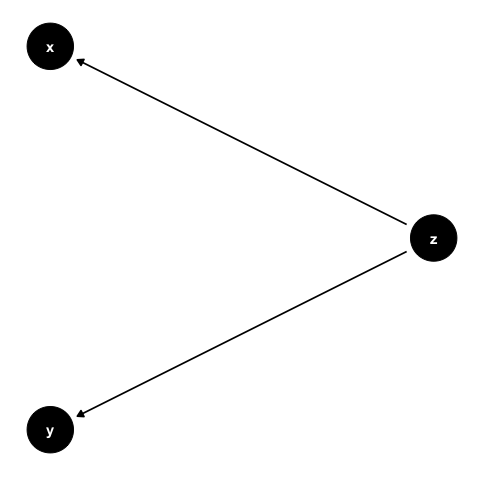A tidy_dagitty object is just a list with a tbl_df, called data, and the dagitty object, called dag:

tidy_dag <- tidy_dagitty(dagified)
str(tidy_dag)
#> List of 2
#>  $data: tibble [4 × 8] (S3: tbl_df/tbl/data.frame) #> ..$ name     : chr [1:4] "z" "z" "x" "y"
#>   ..$x : num [1:4] 23.3 23.3 22.9 23.7 #> ..$ y        : num [1:4] 25.5 25.5 26.6 24.4
#>   ..$direction: Factor w/ 3 levels "<-","->","<->": 2 2 NA NA #> ..$ to       : chr [1:4] "x" "y" NA NA
#>   ..$xend : num [1:4] 22.9 23.7 NA NA #> ..$ yend     : num [1:4] 26.6 24.4 NA NA
#>   ..$circular : logi [1:4] FALSE FALSE FALSE FALSE #>$ dag : 'dagitty' Named chr "dag {\nx [exposure]\ny [outcome]\nz\nz -> x\nz -> y\n}\n"
#>  - attr(*, "class")= chr "tidy_dagitty"

Working with DAGs

Most of the analytic functions in dagitty have extensions in ggdag and are named dag_*() or node_*(), depending on if they are working with specific nodes or the entire DAG. A simple example is node_parents(), which adds a column to the to the tidy_dagitty object about the parents of a given variable:

node_parents(tidy_dag, "x")
#> # A DAG with 3 nodes and 2 edges
#> #
#> # Exposure: x
#> # Outcome: y
#> #
#> # A tibble: 4 × 9
#>   name      x     y direction to     xend  yend circular parent
#>   <chr> <dbl> <dbl> <fct>     <chr> <dbl> <dbl> <lgl>    <fct>
#> 1 z      23.3  25.5 ->        x      22.9  26.6 FALSE    parent
#> 2 z      23.3  25.5 ->        y      23.7  24.4 FALSE    parent
#> 3 x      22.9  26.6 <NA>      <NA>   NA    NA   FALSE    child
#> 4 y      23.7  24.4 <NA>      <NA>   NA    NA   FALSE    <NA>

Or working with the entire DAG to produce a tidy_dagitty that has all pathways between two variables:

bigger_dag <- dagify(y ~ x + a + b,
x ~ a + b,
exposure = "x",
outcome = "y")
#  automatically searches the paths between the variables labelled exposure and
#  outcome
dag_paths(bigger_dag)
#> # A DAG with 4 nodes and 17 edges
#> #
#> # Exposure: x
#> # Outcome: y
#> #
#> # A tibble: 20 × 10
#>    set   name      x     y direction to     xend  yend circular path
#>    <chr> <chr> <dbl> <dbl> <fct>     <chr> <dbl> <dbl> <lgl>    <chr>
#>  1 1     a      17.3  19.2 ->        x      16.8  18.2 FALSE    <NA>
#>  2 1     a      17.3  19.2 ->        y      17.7  18.2 FALSE    <NA>
#>  3 1     b      17.3  17.2 ->        x      16.8  18.2 FALSE    <NA>
#>  4 1     b      17.3  17.2 ->        y      17.7  18.2 FALSE    <NA>
#>  5 1     x      16.8  18.2 ->        y      17.7  18.2 FALSE    open path
#>  6 1     y      17.7  18.2 <NA>      <NA>   NA    NA   FALSE    open path
#>  7 2     a      17.3  19.2 ->        x      16.8  18.2 FALSE    open path
#>  8 2     a      17.3  19.2 ->        y      17.7  18.2 FALSE    open path
#>  9 2     b      17.3  17.2 ->        x      16.8  18.2 FALSE    <NA>
#> 10 2     b      17.3  17.2 ->        y      17.7  18.2 FALSE    <NA>
#> 11 2     x      16.8  18.2 ->        y      17.7  18.2 FALSE    <NA>
#> 12 2     y      17.7  18.2 <NA>      <NA>   NA    NA   FALSE    open path
#> 13 2     x      16.8  18.2 ->        y      17.7  18.2 FALSE    open path
#> 14 3     a      17.3  19.2 ->        x      16.8  18.2 FALSE    <NA>
#> 15 3     a      17.3  19.2 ->        y      17.7  18.2 FALSE    <NA>
#> 16 3     b      17.3  17.2 ->        x      16.8  18.2 FALSE    open path
#> 17 3     b      17.3  17.2 ->        y      17.7  18.2 FALSE    open path
#> 18 3     x      16.8  18.2 ->        y      17.7  18.2 FALSE    <NA>
#> 19 3     y      17.7  18.2 <NA>      <NA>   NA    NA   FALSE    open path
#> 20 3     x      16.8  18.2 ->        y      17.7  18.2 FALSE    open path

ggdag also supports piping of functions and includes the pipe internally (so you don’t need to load dplyr or magrittr). Basic dplyr verbs are also supported (and anything more complex can be done directly on the data object).

library(dplyr)
#  find how many variables are in between x and y in each path
bigger_dag %>%
dag_paths() %>%
group_by(set) %>%
filter(!is.na(path) & !is.na(name)) %>%
summarize(n_vars_between = n() - 1L)
#> # A tibble: 3 × 2
#>   set   n_vars_between
#>   <chr>          <int>
#> 1 1                  1
#> 2 2                  3
#> 3 3                  3

Plotting DAGs

Most dag_*() and node_*() functions have corresponding ggdag_*() for quickly plotting the results. They call the corresponding dag_*() or node_*() function internally and plot the results in ggplot2.

ggdag_paths(bigger_dag)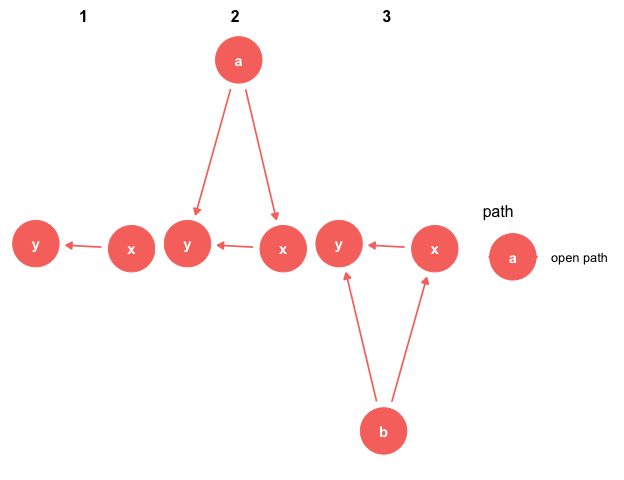ggdag_parents(bigger_dag, "x")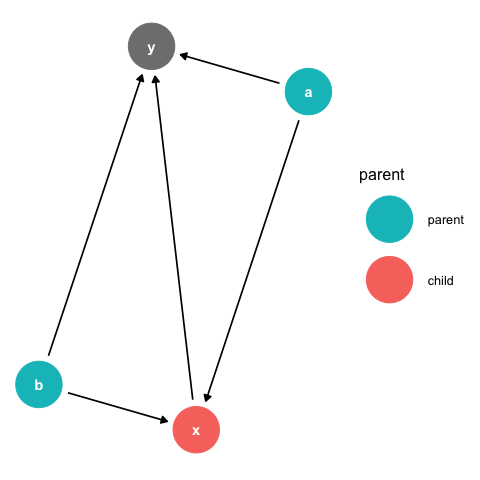#  quickly get the miniminally sufficient adjustment sets to adjust for when
#  analyzing the effect of x on y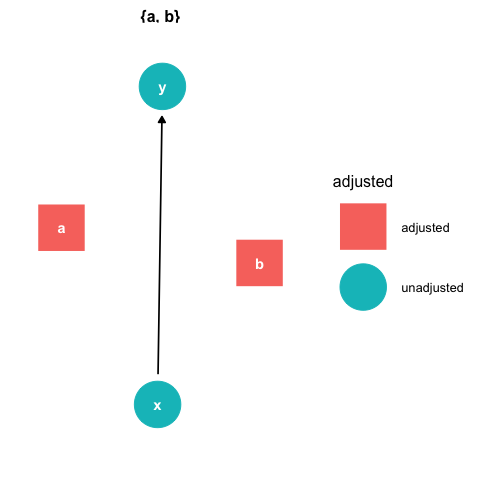Plotting directly in ggplot2

ggdag() and friends are, by and large, fairly thin wrappers around included ggplot2 geoms for plotting nodes, text, and edges to and from variables. For example, ggdag_parents() can be made directly in ggplot2 like this:

bigger_dag %>%
node_parents("x") %>%
ggplot(aes(x = x, y = y, xend = xend, yend = yend, color = parent)) +
geom_dag_point() +
geom_dag_edges() +
geom_dag_text(col = "white") +
theme_dag() +
scale_color_hue(breaks  = c("parent", "child")) #  ignores NA in legend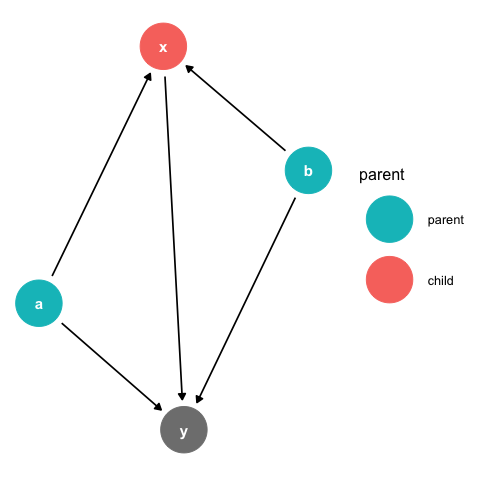The heavy lifters in ggdag are geom_dag_node()/geom_dag_point(), geom_dag_edges(), geom_dag_text(), theme_dag(), and scale_adjusted(). geom_dag_node() and geom_dag_text() plot the nodes and text, respectively, and are only modifications of geom_point() and geom_text(). geom_dag_node() is slightly stylized (it has an internal white circle), while geom_dag_point() looks more like geom_point() with a larger size. theme_dag() removes all axes and ticks, since those have little meaning in a causal model, and also makes a few other changes. expand_plot() is a convenience function that makes modifications to the scale of the plot to make them more amenable to nodes with large points and text scale_adjusted() provides defaults that are common in analyses of DAGs, e.g. setting the shape of adjusted variables to a square.

geom_dag_edges() is also a convenience function that plots directed and bi-directed edges with different geoms and arrows. Directed edges are straight lines with a single arrow head, while bi-directed lines, which are a shorthand for a latent parent variable between the two bi-directed variables (e.g. a <- L -> b), are plotted as an arc with arrow heads on either side.

You can also call edge functions directly, particularly if you only have directed edges. Much of ggdag’s edge functionality comes from ggraph, with defaults (e.g. arrow heads, truncated lines) set with DAGs in mind. Currently, ggdag has four type of edge geoms: geom_dag_edges_link(), which plots straight lines, geom_dag_edges_arc(), geom_dag_edges_diagonal(), and geom_dag_edges_fan().

dagify(y ~ x,
m ~ x + y) %>%
ggplot(aes(x = x, y = y, xend = xend, yend = yend)) +
geom_dag_point() +
geom_dag_edges_arc() +
geom_dag_text() +
theme_dag()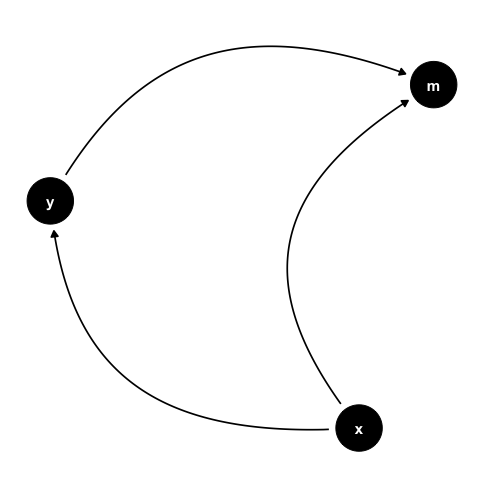If you have bi-directed edges but would like to plot them as directed, node_canonical() will automatically insert the latent variable for you.

dagify(y ~ x + z,
x ~~ z) %>%
node_canonical() %>%
ggplot(aes(x = x, y = y, xend = xend, yend = yend)) +
geom_dag_point() +
geom_dag_edges_diagonal() +
geom_dag_text() +
theme_dag()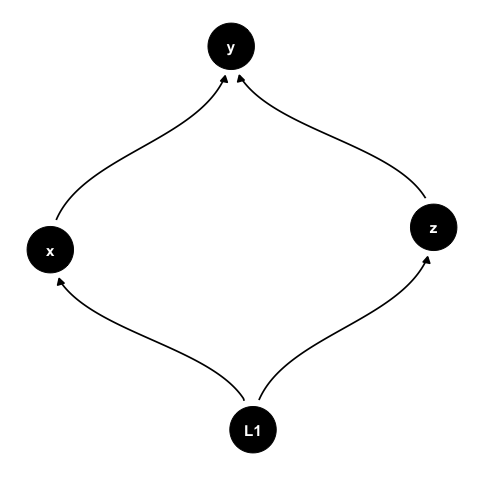There are also geoms based on those in ggrepel for inserting text and labels, and a special geom called geom_dag_collider_edges() that highlights any biasing pathways opened by adjusting for collider nodes. See the vignette introducing DAGs for more info.